﻿ 基于二阶相似度的即时学习软测量建模方法
«上一篇文章快速检索 高级检索

 智能系统学报2020, Vol. 15Issue (5): 910-918  DOI: 10.11992/tis.2018090400

### 引用本文QI Cheng, SHI Xudong, XIONG Weili. A just-in-time learning soft sensor modeling method based on the second-order similarity[J]. CAAI Transactions on Intelligent Systems, 2020, 15(5): 910-918. DOI: 10.11992/tis.201809040.### 文章历史

1. 江南大学 物联网工程学院，江苏 无锡 214122;
2. 江南大学 轻工过程先进控制教育部重点实验室，江苏 无锡 214122

A just-in-time learning soft sensor modeling method based on the second-order similarity
QI Cheng 1, SHI Xudong 1, XIONG Weili 1,21. School of Internet of Things Engineering, Jiangnan University, Wuxi 214122, China;
2. Key Laboratory of Advanced Process Control for Light Industry Jiangnan University, Ministry of Education, Wuxi 214122, China
Abstract: Aiming at the indirect accuracy reduction caused by the frequency reduction of just-in-time (lazy) learning model, a second-order similarity just-in-time learning method is proposed. This method takes into account the overall distribution characteristics of the sample set, establishes a second-order similarity criterion based on the traditional first-order similarity criterion, and uses a second-order similarity sample with most of the same neighbors as the test sample to establish the model at the current time. At the same time, the cumulative similarity factor is used to determine the sample size when the local model is established, and the similarity threshold is used to determine whether the model needs to be rebuilt at this time. This method has been effectively validated in the prediction experiment of the product concentration in the fermentation process of penicillin.
Key words: just-in-time learning    update frequency    second-order similarity    similarity criterion    first-order similarity    local model    cumulative similarity factor    similarity threshold

1 基于二阶相似度的即时学习方法 1.1 传统的即时学习方法

JITL是根据相似输入产生相似输出的思想，假设有N个历史训练样本的数据集 ${ X}\{ {{ x}_i} \in {{\rm R}^m},$ $i = 1,2 \cdots N\}$ ，其中m表示输入数据的维度。若预测某一时刻待测样本 ${{{x}}_q}$ ，JITL的方式是在历史集中选择与 ${{{x}}_q}$ 拥有最高相似性的一组样本来构建当前的局部模型，同时采用该模型预测 ${{{x}}_q}$ 。相似度准则是JITL的核心，现对常用的FOS进行描述：

 ${\rm SI} = \gamma \sqrt {\exp ( - {d^2})} + (1 - \gamma ) \cos\; \theta$ (1)

 $d{\rm{ = }}{\left\| {{{ x}_q} - {{ x}_i}} \right\|_2}$ (2)
 $\theta = {\rm{arc}}\cos \frac{{{ x}_q^{\rm{T}}{{ x}_i}}}{{{{\left\| {{{ x}_q}} \right\|}_2} \cdot {{\left\| {{{ x}_i}} \right\|}_2}}}$ (3)
1.2 二阶相似度策略 1.2.1 二阶相似度的定义

SOS定义为：如果两样本的一阶相似样本大多相同，那么认为两样本拥有较高的二阶相似性。FOS准则仅关注单独两样本之间的相似度，忽略了样本集总体的二阶相似性。如图1所示，数据1与2的FOS很高，数据1和8的近邻大多数相同，因此，二者的SOS很高，同理数据2与8的二阶相似性也较高。假设当前到来待测数据1，若采用FOS准则选取相似集，仅仅选择到数据2；但改用SOS策略时，不仅可以选择到数据2，还能选择到数据8。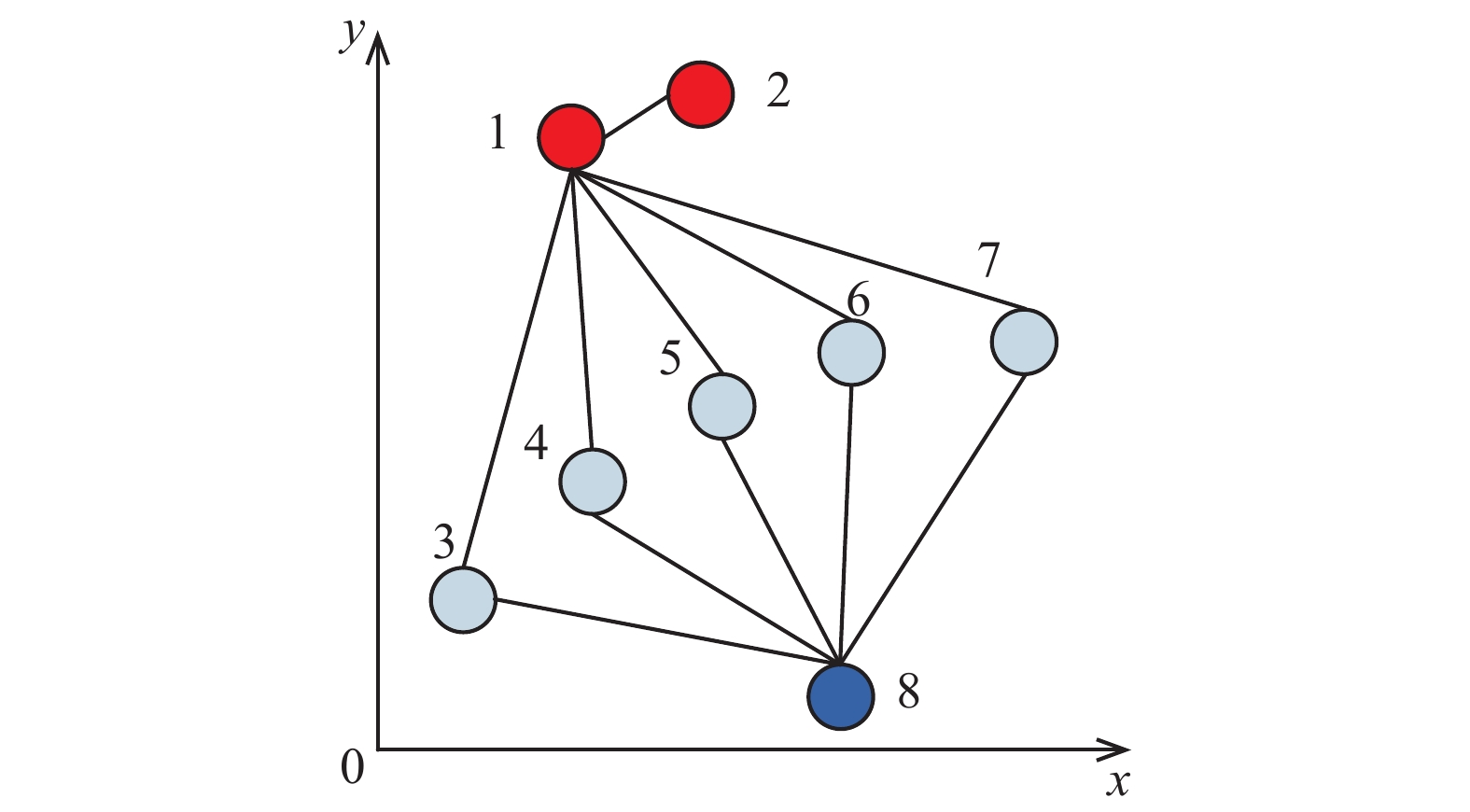Download: 图 1 二维空间例子 Fig. 1 Two-dimensional space example

SOS算法的伪代码如下：

for $i = 1:N$

for $j = 1:N$

end for

end for

for $i = 1:N$

通过式(1)计算测试样本 ${{ x}_q}$ 和训练样本 ${{ x}_i}$ 之间的FOS

end for

for $i = 1:N$

计算 ${{ \bar{ i}}_q}$ ${{ \bar{ i}}_i}$ 拥有相同索引值的个数

end for

1.2.2 SOS的特性分析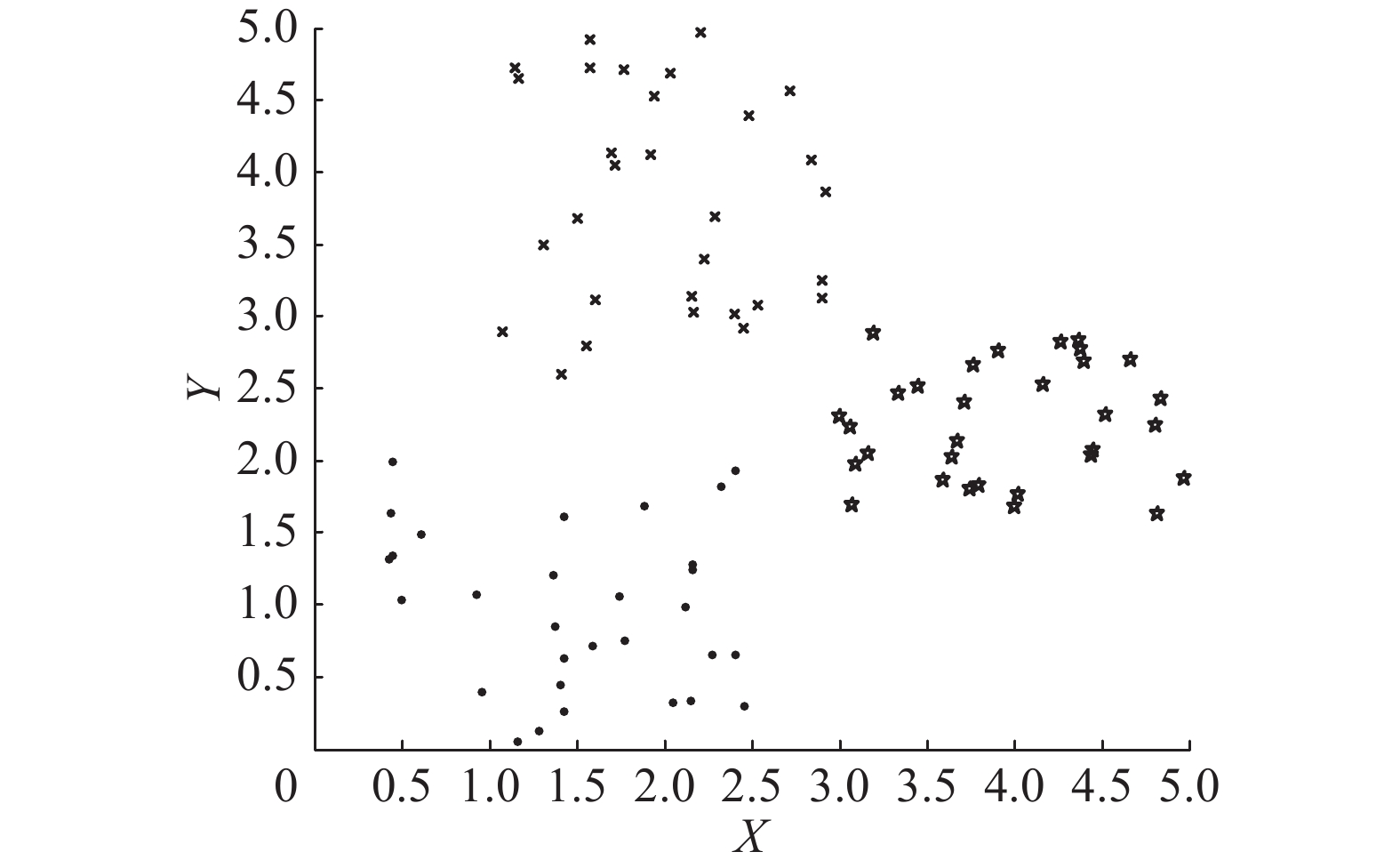Download: 图 2 产生的3类训练样本集 Fig. 2 Three types of training sample sets generated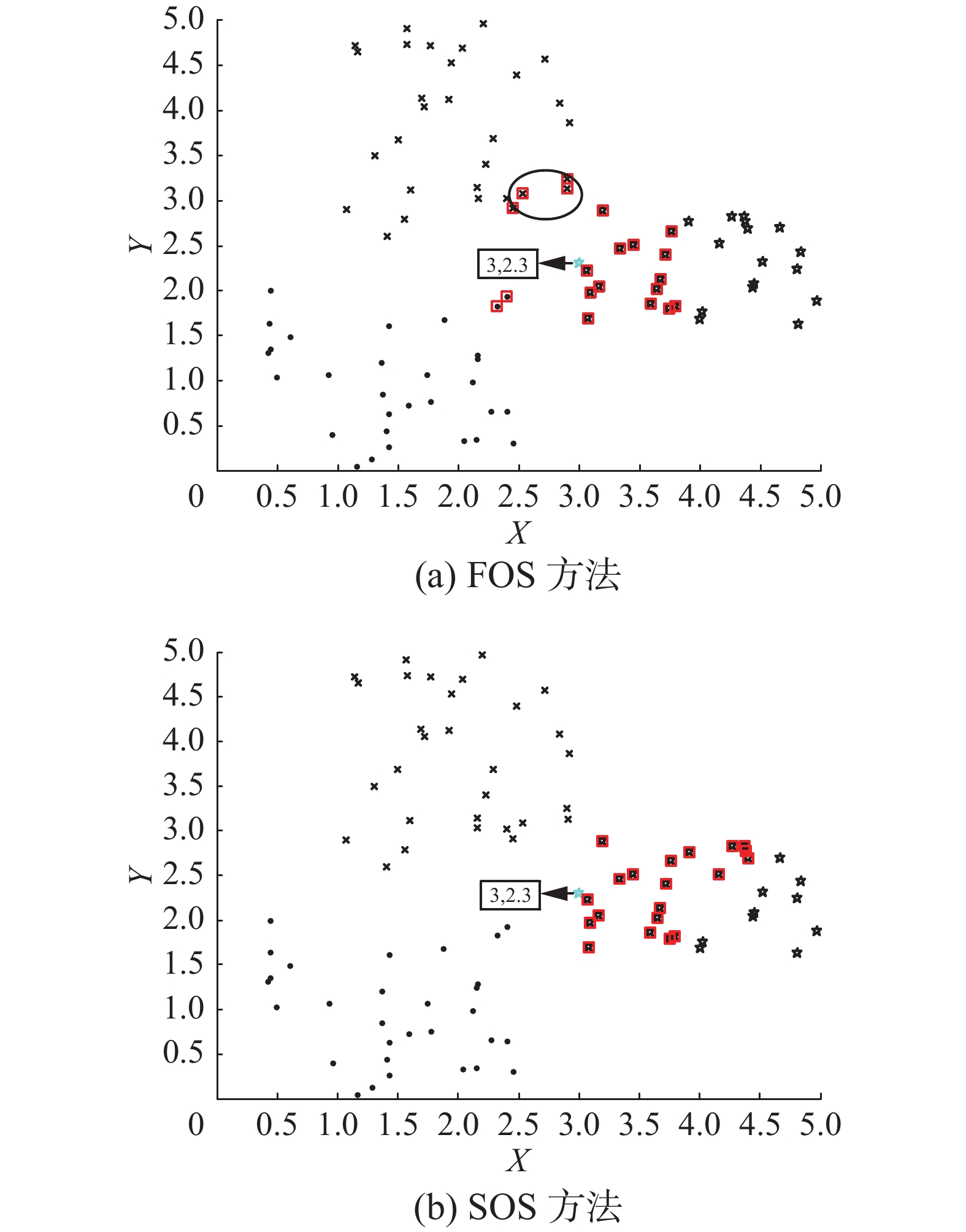Download: 图 3 FOS方法与SOS方法的区别 Fig. 3 The difference between FOS and SOS

1.3 累计相似度因子

 ${s_k} = \frac{{\displaystyle\sum\limits_{i = 1}^k {{s_{qi}}} }}{{\displaystyle\sum\limits_{i = 1}^N {{s_{qi}}} }},0 \leqslant k \leqslant N$ (4)

2 自适应更新机制的GPR模型 2.1 模型更新机制

1) 若当前为q时刻，经过计算且模型需要更新，当来到新的测试数据 ${{ x}_q}$ ，采用1.2节的方法从历史样本集中选择与 ${{ x}_q}$ SOS最高的一批数据建立当前的GPR模型，同时估计 ${{ x}_q}$ ，并将SOS最高的值乘以某一数值(参数)用于判断之后时刻模型是否需要更新的THR。

2) 当 $q + 1$ 时刻数据 ${{ x}_{q + 1}}$ 到来，计算其和 ${{ x}_{q }}$ 之间的SOS，同时与THR比较，若小于THR，则更新模型进行估计，同时更新相似度阈值，否则，认为当前工况变化较小，仍采用q时刻的局部模型对 ${{ x}_{q + 1}}$ 进行估计。

3) 如果 $q + 1$ 未更新模型，在 $q + 2$ 时刻，计算测试数据 ${{ x}_{q + 2}}$ 和前一模型更新时刻数据 ${{ x}_{q }}$ 之间的SOS，其他的判断方式与 ${{ x}_{q + 1}}$ 相同。

2.2 GPR建模方法

GPR是一种非参数概率模型，已经成为机器学习领域的一种重要的建模方法，并在近些年来得到广泛关注与应用[15-17]，其基本原理描述如下。

 ${y_i} = f({{ x}_i}) + \varepsilon$ (5)

 ${y_q}({{ x}_q}) = {{ c}^{\rm T}}({{ x}_q}){{ C}^{ - 1}}{ Y}$ (6)
 $\sigma _{{y_q}}^2({{ x}_q}) = c({{ x}_q},{{ x}_q}) - {{ c}^{\rm T}}({{ x}_q}){{ C}^{ - 1}}{ c}({{ x}_q})$ (7)

GPR有不同的协方差函数可以选择，本文选取径向基协方差函数，其详细描述如下：

 $c({{ x}_i},{{ x}_j}) = v\exp \left[ { - \frac{1}{2}\sum\limits_{{{t}} = 1}^m {{\omega _t}{{({{ x}_{it}} - {{ x}_{jt}})}^2}} } \right] + \sigma _N^2{\delta _{ij}}$ (8)

 $L({ \theta }) = - \frac{1}{2}\log (\det ({ C})) - \frac{1}{2}{{ Y}^{\rm T}}{{ C}^{ - 1}}{ Y} - \frac{N}{2}\log (2\pi )$ (9)

3 基于二阶相似度的即时学习建模步骤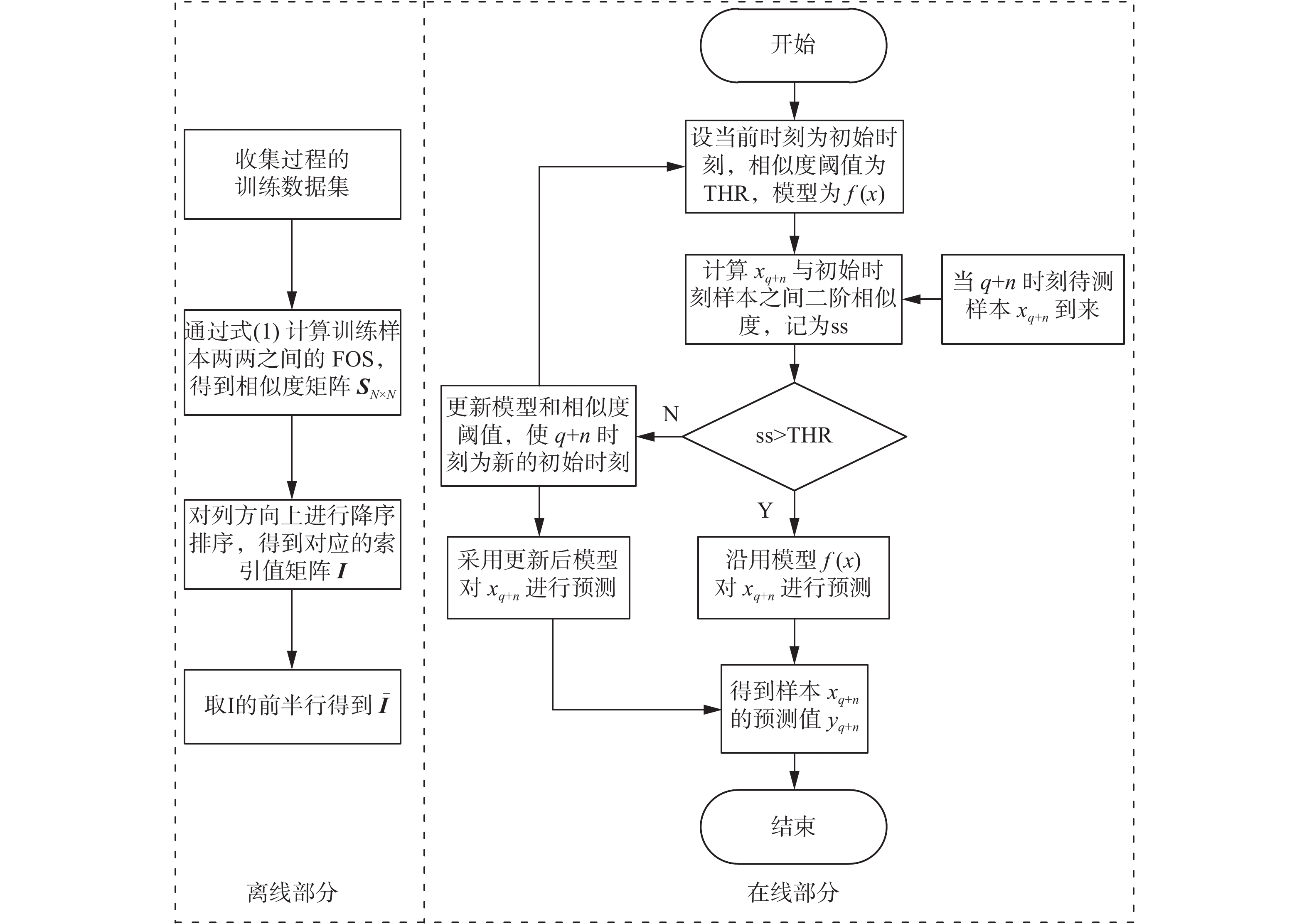Download: 图 4 基于SOS-JITL方法的建模流程图 Fig. 4 Modeling flow chart based on SOS-JITL method

1）采用式(1)计算历史数据相互间的FOS，得到相似度矩阵，同时将其降序排序返回索引值矩阵 ${{I}}$

2）假如当前q时刻模型需要更新，针对待测数据xq，计算其与历史数据之间的FOS，并返回索引值向量 ${{ i}_q}$

3）通过1.2节计算 ${{ \bar{ i}}_q}$ ${{ \bar{ i}}_i}$ 间的SOS，同时采用累积相似度因子自适应选取与 ${{ x}_q}$ 近邻最多的一批数据建立此刻的GPR模型，并估计 ${{ x}_q}$ 同时更新模型的THR。

4）若 $q + 1$ 时刻测试数据 ${{ x}_{q + 1}}$ 到来，计算其和当前模型更新之初时刻数据 ${{ x}_q}$ 间的SOS，并与THR比较，若小于THR，如步骤3）更新模型和THR，并采用新模型估 ${{ x}_{q + 1}}$ ，同时将 $q + 1$ 时刻设置为模型更新之初时刻；否则，仍采用q时刻模型估计 ${{ x}_{q + 1}}$

5)对于 $q + n$ 时刻的待测数据 ${{ x}_{q + n}}$ ，重复步骤4），可得估计值 ${{ y}_{q + n}}$

4 实验仿真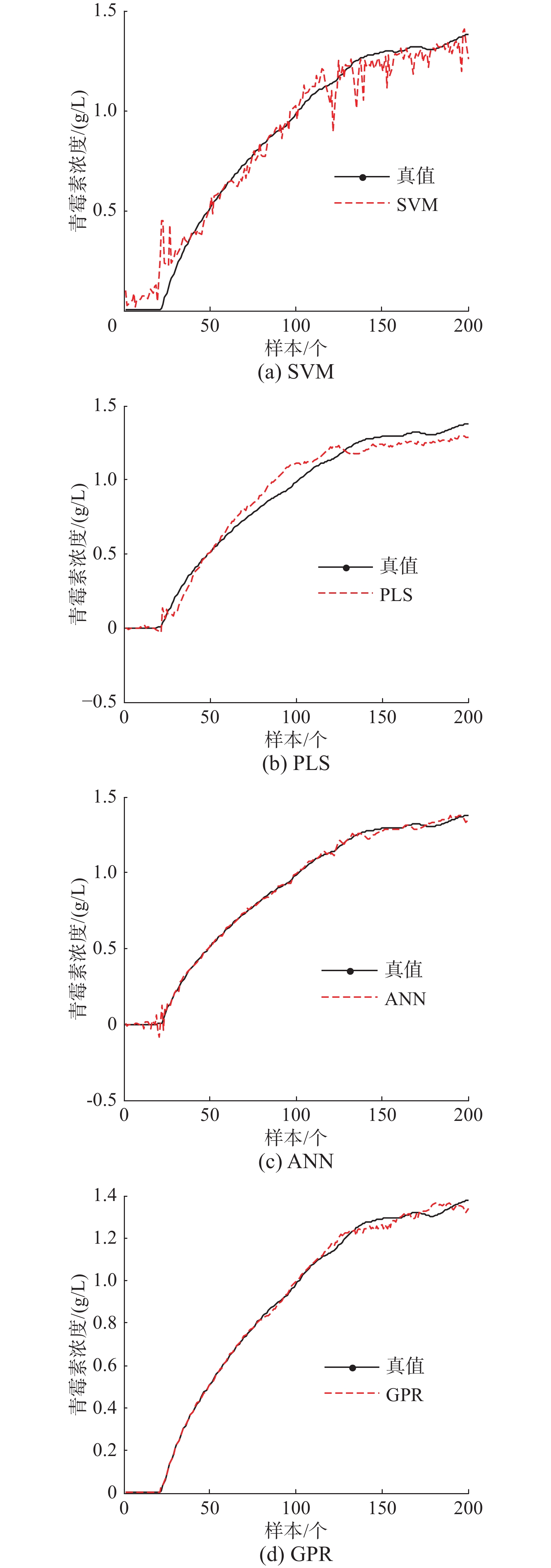Download: 图 5 4种基本建模方法的预测曲线 Fig. 5 Prediction curve of the four basic modeling methods

 ${\rm{RMSE}}{ = }\sqrt {\frac{{\displaystyle\sum\limits_{j = 1}^M {{{({y_j} - y_j^ * )}^2}} }}{M}}$ (10)表 1 4种基本建模方法的RMSE Tab.1 RMSE of the four basic modeling methods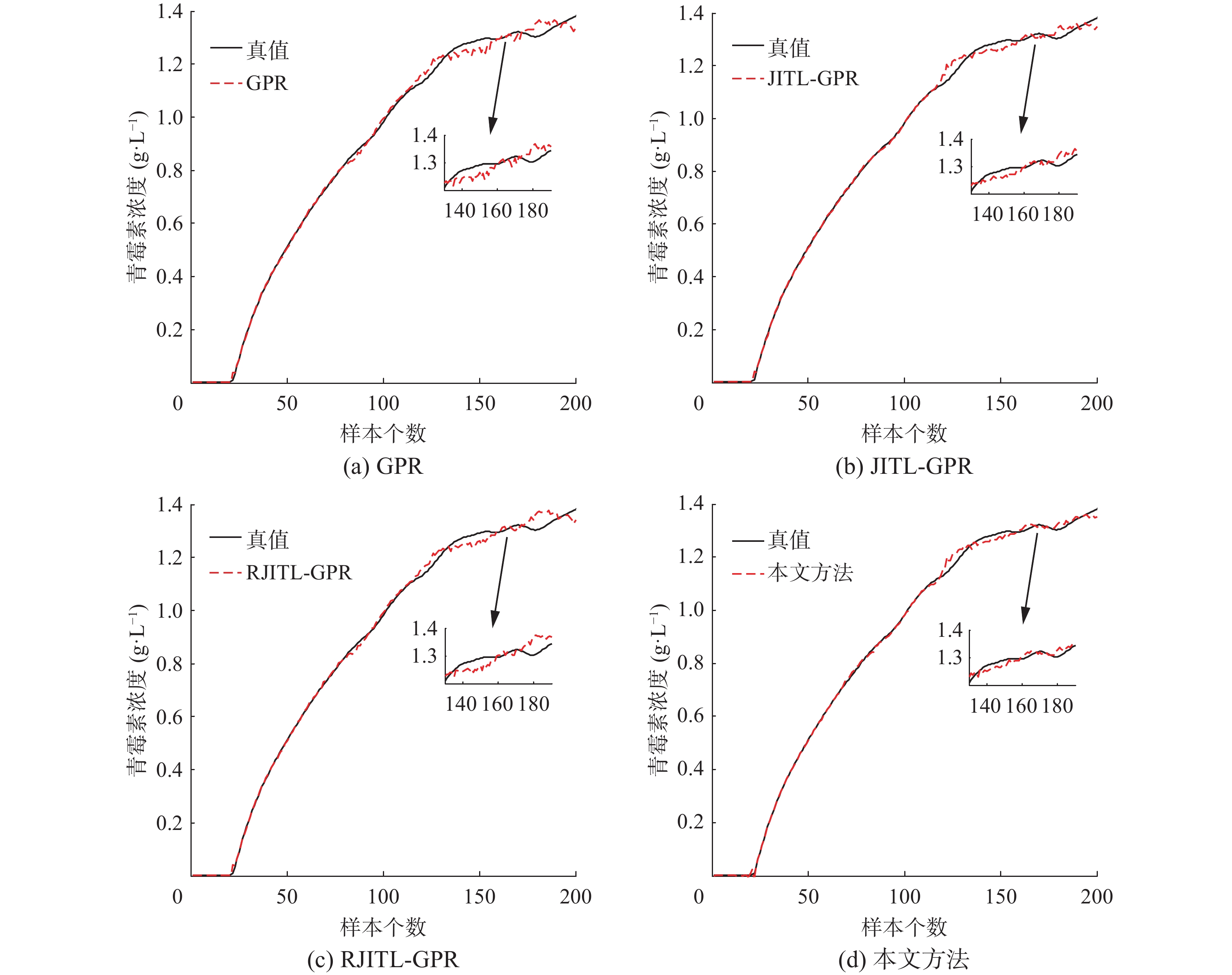Download: 图 6 4种方法的预测曲线 Fig. 6 Prediction curve of the four methods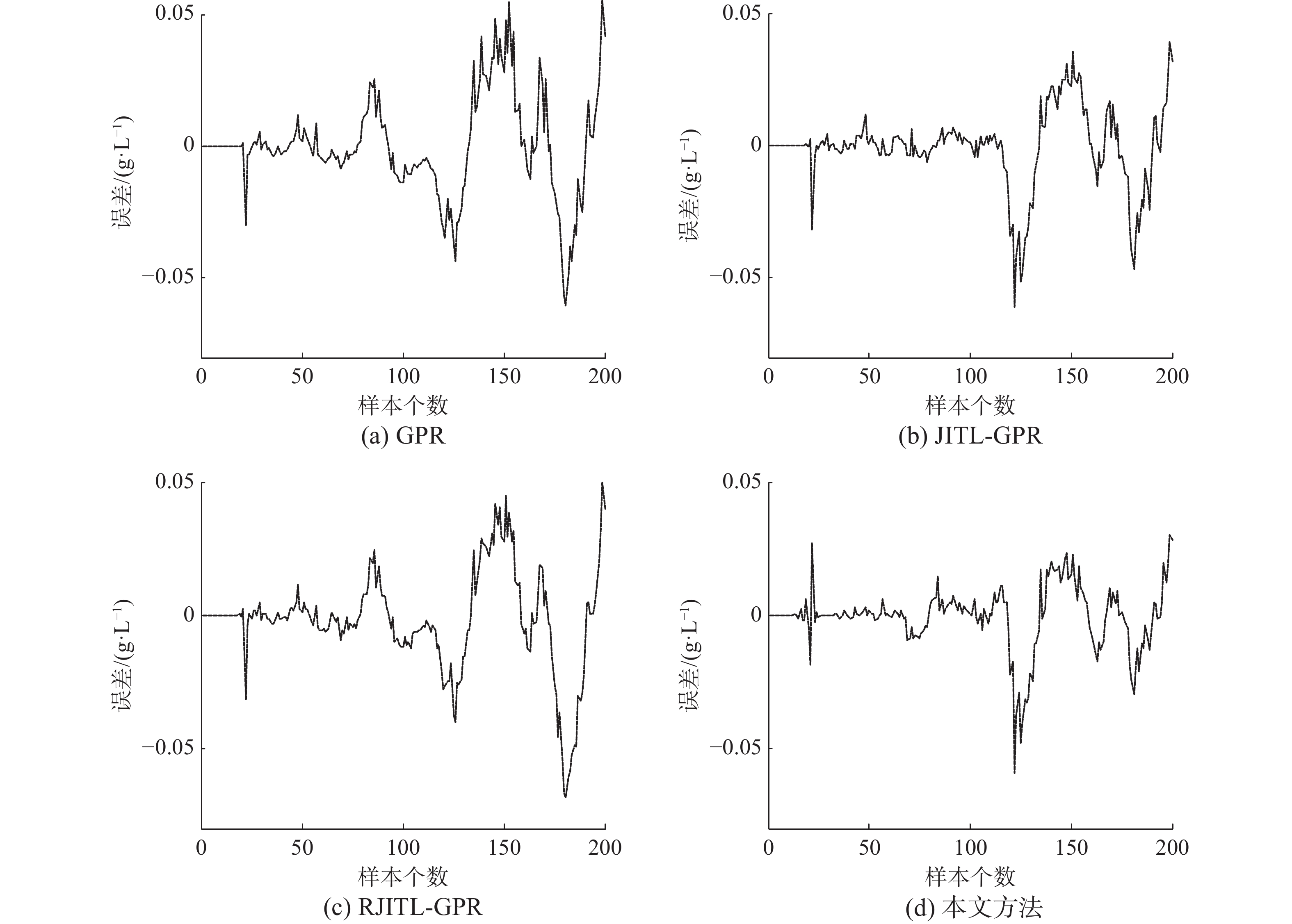Download: 图 7 4种方法的预测误差曲线 Fig. 7 Prediction error curve of the four methods表 2 4种方法的RMSE和建模时间 Tab.2 RMSE and modeling time of the four methods
5 结束语

  汤健, 柴天佑, 刘卓, 等. 基于更新样本智能识别算法的自适应集成建模[J]. 自动化学报, 2016, 42(7): 1040-1052. TANG Jian, CHAI Tianyou, LIU Zhuo, et al. Adaptive ensemble modelling approach based on updating sample intelligent identification[J]. Acta automatica sinica, 2016, 42(7): 1040-1052. (0)  ZHENG Junhua, SONG Zhihuan. Semisupervised learning for probabilistic partial least squares regression model and soft sensor application[J]. Journal of process control, 2018, 64: 123-131. DOI:10.1016/j.jprocont.2018.01.008 (0)  LI Han, YOU Shijun, ZHANG Huan, et al. Analyzing the impact of heating emissions on air quality index based on principal component regression[J]. Journal of cleaner production, 2018, 171: 1577-1592. DOI:10.1016/j.jclepro.2017.10.106 (0)  ZHENG Jianqiao, WANG Hongfang, ZHOU Hongpeng, et al. A using of just-in-time learning based data driven method in continuous stirred tank heater[C]//Proceedings of the 7th International Conference on Intelligent Control and Information Processing. Siem Reap, Cambodia, 2016: 98-104. (0)  PENG Xin, TANG Yang, HE Wangli, et al. A just-in-time learning based monitoring and classification method for hyper/hypocalcemia diagnosis[J]. IEEE/ACM transactions on computational biology and bioinformatics, 2018, 15(3): 788-801. DOI:10.1109/TCBB.2017.2655522 (0)  YIN Shen, GAO Huijun, QIU Jianbin, et al. Fault detection for nonlinear process with deterministic disturbances: a just-in-time learning based data driven method[J]. IEEE transactions on cybernetics, 2017, 47(11): 3649-3657. DOI:10.1109/TCYB.2016.2574754 (0)  CHEN Kun, LIU Yi. Adaptive weighting just-in-time-learning quality prediction model for an industrial blast furnace[J]. ISIJ international, 2017, 57(1): 107-113. DOI:10.2355/isijinternational.ISIJINT-2016-292 (0)  NIU Dapeng, GAO Huiyuan, LIU Yuanqing. Modeling of penicillin fermentation process based on FCM and improved Just-in-Time learning algorithm[C]//Proceedings of the 36th Chinese Control Conference. Dalian, China, 2017: 10328-10332. (0)  GE Zhiqiang, SONG Zhihuan. A comparative study of just-in-time-learning based methods for online soft sensor modeling[J]. Chemometrics and intelligent laboratory systems, 2010, 104(2): 306-317. DOI:10.1016/j.chemolab.2010.09.008 (0)  张宏伟, 李鹏飞, 景军锋, 等. 基于即时学习的软测量建模实时性改进[J]. 西安工程大学学报, 2014, 28(6): 750-754. ZHANG Hongwei, LI Pengfei, JING Junfeng, et al. A real-time performance improvement strategy of Just-In-Time-Learning based on soft sensor[J]. Journal of Xi’an Polytechnic University, 2014, 28(6): 750-754. DOI:10.3969/j.issn.1674-649X.2014.06.022 (0)  牛大鹏, 刘元清. 基于改进即时学习算法的湿法冶金浸出过程建模[J]. 化工学报, 2017, 68(7): 2873-2879. NIU Dapeng, LIU Yuanqing. Modeling hydrometallurgical leaching process based on improved just-in-time learning algorithm[J]. CIESC journal, 2017, 68(7): 2873-2879. (0)  CRIBBIN T. Discovering latent topical structure by second-order similarity analysis[J]. Journal of the American society for information science and technology, 2011, 62(6): 1188-1207. DOI:10.1002/asi.v62.6 (0)  刘毅, 金福江, 高增梁. 时变过程在线辨识的即时递推核学习方法研究[J]. 自动化学报, 2013, 39(5): 602-609. LIU Yi, JIN Fujiang, GAO Zengliang. Online identification of time-varying processes using just-in-time recursive kernel learning approach[J]. Acta automatica sinica, 2013, 39(5): 602-609. (0)  LIU Yi, GAO Zengliang. Industrial melt index prediction with the ensemble anti-outlier just-in-time Gaussian process regression modeling method[J]. Journal of applied polymer science, 2015, 132(22): 41958. (0)  WANG Haijun, GAO Xinbo, ZHANG Kaibing, et al. Single image super-resolution using Gaussian process regression with dictionary-based sampling and student-t likelihood[J]. IEEE transactions on image processing, 2017, 26(7): 3556-3568. (0)  HAN Jianan, ZHANG Xiaoping, WANG Fang. Gaussian process regression stochastic volatility model for financial time series[J]. IEEE journal of selected topics in signal processing, 2016, 10(6): 1015-1028. DOI:10.1109/JSTSP.2016.2570738 (0)  XIONG Weili, SHI Xudong. Soft sensor modeling with a selective updating strategy for Gaussian process regression based on probabilistic principle component analysis[J]. Journal of the franklin institute, 2018, 355(12): 5336-5349. DOI:10.1016/j.jfranklin.2018.05.017 (0)  何志昆, 刘光斌, 赵曦晶, 等. 高斯过程回归方法综述[J]. 控制与决策, 2013, 28(8): 1121-1129, 1137. HE Zhikun, LIU Guangbin, ZHAO Xijing, et al. Overview of Gaussian process regression[J]. Control and decision, 2013, 28(8): 1121-1129, 1137. (0)  YU Jie. Multiway Gaussian mixture model based adaptive kernel partial least squares regression method for soft sensor estimation and reliable quality prediction of nonlinear multiphase batch processes[J]. Industrial & engineering chemistry research, 2012, 51(40): 13227-13237. (0)  LIU Ziwei, GE Zhiqiang, CHEN Guangjie, et al. Adaptive soft sensors for quality prediction under the framework of Bayesian network[J]. Control engineering practice, 2018, 72: 19-28. DOI:10.1016/j.conengprac.2017.10.018 (0)